n queen topics
Home
Group actions
Search algorithm
Use symmetry, regular NQ problem
Evolution trees
Special properties
Necklaces
Conflicts
Conflict tables
World records
Tables of counting results
Images for n=8
Torus images for n=13
Other pages to the n queens problem
Bibliografy

Other themes
Primes from sums of factors
Primes from wrong binomic formula
Numbers by primes distribution

German

# First solution in alphabetic order, for the n-queens problem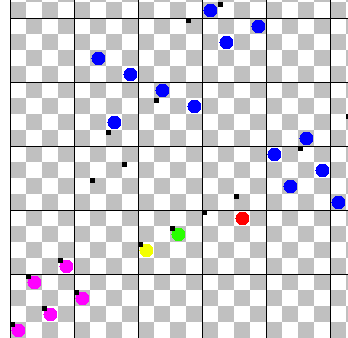The first n-queens solution on the 21 x 21 board.

The regular n-queens problem has very many solutions, for larger board sizes.

Therefore, it seems easy to find the first of the solutions. But it is not easy at all, it is hard.

Of course, we have to define first what we mean with "first solution". It means that we use an alphabetic order among the solutions. We enumerate the positions of queens on the board row by row, and write down the column of the queen in the actual row. Up to n = 26, we replace the number of the column by the corresponding letter of the alphabet, and get a (generalised) word of n letters. The order of solutions is then exactly as the order of the corresponding words would be in a lexikon. We call this "alphabetic order".

The reason why it is hard is that the solutions are not distributed uniformly over the whole area of possible solutions. The following images show by the color of a cell how many solutions have a queen on this cell. Interpretation is like a temperature chart of the weather forecast: blue means very cold, only few solutions, green not so cold, but under median value, yellow means warm, number of solutions over median value, and red means a hot area, many solutions. The four colors change their intensity, for blue and green, high intensity indicates less solutions, and for yellow and red, more intensity means more solutions. If there are no solutions at all having a queen on the cell, it is left empty.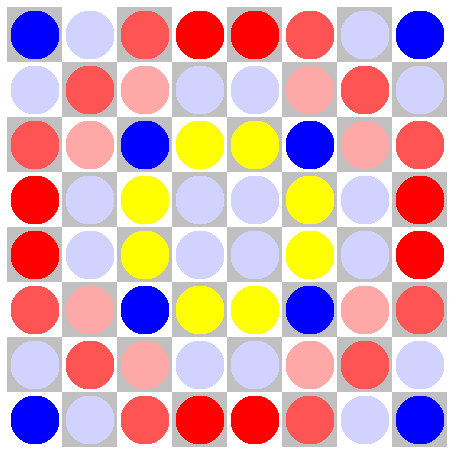Weather chart, nr solution per cell, for empty N8 board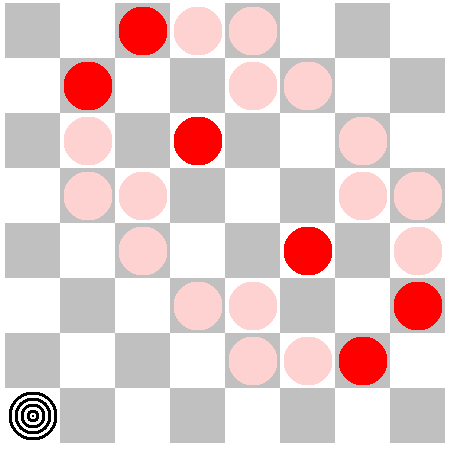Weather chart, nr solution per cell, N8 board, queen on 0/0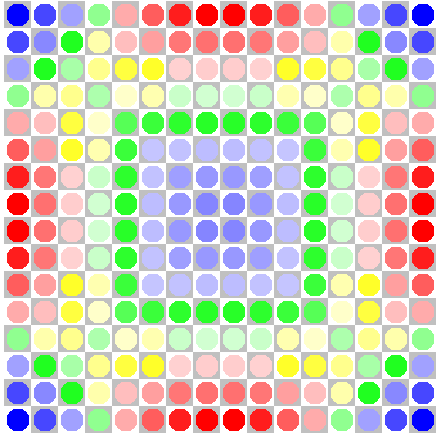Weather chart, nr solution per cell, for empty N16 boardWeather chart, nr solution per cell, N16 board, queen on 0/0

The numbers in the bottom row for N8 are now: 4 / 8 / 16 / 18, and for the next rows 8 / 16 / 14 / 8, 16 / 14 / 4 / 12, and 18 / 8 / 12 / 8. The ratio of the best (in the middle) to the worst (at the corner) is 4.5.

The numbers in the bottom row for N16 are now: 436,228 / 569,531 / 736,363 / 892,999 / 1,050,762 / 1,160,280 / 1,249,262 / 1,290,831. With larger n, the distribution should be more uniform; but the ratio best to worst is still 2.96.

Looking at these images, one can see that the first queen on the corner field gives only few possibilities for solutions. That is bad for searching the first solution. Despite there are few solutions, one has to check many fields which do not lead to solutions. Even more, with the images having a queen on cell 0/0, we see on the 8 by 8 board, that there exist no solutions with the second queen on 2/1 or 3/1. Queens on 0/1 or 1/1 would break the rules of quees, so it is no surprise that these cells are empty. But the other two cells have no obvious reason to be un-eligable.

The corresponding image for N16 shows that queens on 2/1 or 3/1 are possible; but again, there are few solutions. There are 16,346 possibilities for 2/1, compared to 44,128 on 8/1.

Continuing on this way, we get the next images: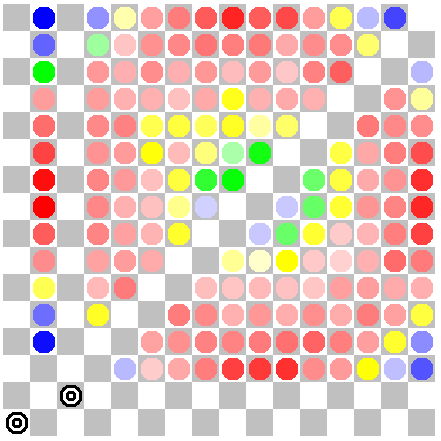Nr solution per cell, for N16 with 2 queens in minimal position; total 16,364 solutions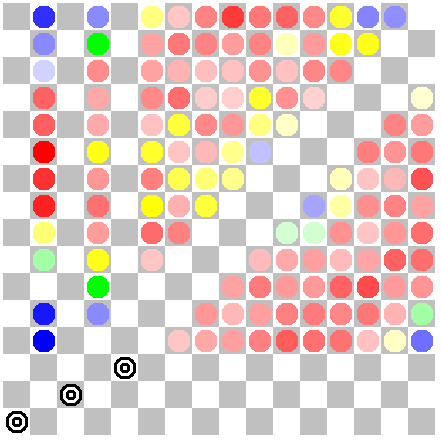Nr solution per cell, for N16 with 3 queens in minimal position; total 819 solutions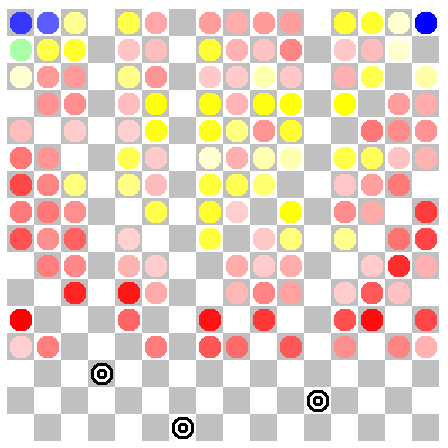Nr solution per cell, for N16 with 3 queens in random position; total 13,547 solutions

I did a similar check on the 24 by 24 board, with seven preset queens. If the preset queens are in minimal position, there are 26 solution, for another position of the preset queens, chosen at random, I got a number of 2,849,191 solutions. This demonstrates how sparse the solutions are in the area of minimal first queens.

# Found first solutions

Today, the first solutions are known for sizes up to 61; until 2017, it was only to size 55. You can look at them in one big gif image which shows them in a loop.

Most of the search was done by Colin Pearson; he maintains a page for this topic. Also Wolfram Schubert and the author (M.Engelhardt) helped with the search.

In the fall of 2017, Matteo Fischetti and Domenico Salvagnin of Univeristy of Padua took up the problem. They use Integer Linear Programming (ILP) and found all the smallest solutions from size 56 to 61, all odd sizes under 74, and also 77, 79, 85, 91, 93, 97, 101, 103, 109, and 115.

It is astonishing that the search effort is not directly coupled to the size; for instance, size 48 needs more effort than every size between 49 and 55, inclusive. It seems this is caused by the divisibility of the number, but we have no further explanation. Up to 2017, we thought it would be a big challenge to find the first solution for the 60 by 60 board, but that is no longer true. Size 48 is still the size needing most effort. A paper on this topic was written by Matteo Fischetti and Domenico Salvagnin, Chasing First Queens by Integer Programming.

# The greedy sequence

Imagine an infinite chessboard; this means, rows and columns start by 1, but do not end at a given number. The question of the smallest nqueen solution can be posed also for this board. The sequence of the columns of the smallest solution is the greedy sequence; it is contained in the encyclopedia of integer sequences, under A065188. Let us call the column of the queen in row k as g(k). (g as abbreviation for "greedy").

The algorithm to find the values of the sequence is very simple: start with the first queen at 1/1. Then go up row by row, and take the first available field. Then, the column is "consumed" or "eaten up"; this is the reason for the name of the sequence, I think.

The greedy sequence has an intresting property: it is a permutation of the natural numbers. That means both that the same column is never used twice (the mapping is injective), and every column is consumed, sooner or later (mapping is surjective); the latter seems evident, but perhaps not so easy to prove.

As the greedy sequence is a permutation, one can ask how many columns are used without hole when the k-th row got its queen. Let me call this number f(k). (f as abbreviation for "filled"). It is clear that f is a monotonic mapping. My computations show that k / f(k) converges to the Fibonacci number, i.e. (sqrt(5) - 1) / 2 = 1.618034. Up to now, I have no proof of it.

A small table of values which caused me to think that k / f(k) converges to the Fibonacci number; the last column (Delta) gives the difference to it.

 k g(k) f(k) k / f(k) Delta 50 32 32 1.5625 0.055534 100 162 62 1.639344 -0.02131 200 323 124 1.612903 0.005131 500 310 311 1.607717 0.010317 1000 618 619 1.615509 0.002525 2000 3236 1236 1.618123 -0.000089 5000 8089 3092 1.617076 0.000958 9999 16179 6179 1.618223 -0.000189

# Other start values for the greedy sequence

One can think of a greedy sequence with some different start values; i.e. the algorithm is the same, the sequence is still "greedy", but the first values are different.

Also in this case, the limit value is the same, appearently. This led me to the question if the sequence itself also becomes the same. To say it in a more formal way: if h(k) is the greedy sequence with different start values, if there exists some natural number m such that g(k) = h(k), for all k > m. I was a bit disappointed that I could not find this, except for trivial constellations. An example for a trivial constellation are the start values h(1) = 2, h(2) = 4, h(3) = 1, h(4) = 3, h(5) = 5. The footprint of the first 5 queens on columns and on diagonals of both directions is exactly the same, in this case; hence, there is no difference in the following terms.

In some cases, I had long areas of k where g(k) = h(k); but then, there was again a difference.

Prepared by Matthias Engelhardt

last change: 2018-01-28